HomePreschoolerLearning & EducationBefore, After And In Between Numbers For Preschoolers To Improve Math Skills

# Before, After And In Between Numbers For Preschoolers To Improve Math Skills

After learning basic counting, children must be introduced to the concept of between, after and before numbers. It not only improved their counting skills but also helped them with problem-solving skills. Learning to identify the before, after and in-between numbers concept hugely benefit kids in understanding the concepts like sequence and patterns.

In this article, we have covered the mathematical concept of between, before and after numbers for kindergarten kids. We have also mentioned some activities at the end to help children learn this concept easily. Let’s begin!

## At What Age Will Your Child Learn About Before, After And In Between Numbers?

Most children can understand the concept of numbers by the age of 2-4. Therefore, at this age, your child can learn about before, after and between numbers in mathematics.

## How To Teach The Concept Of Before, After And In Between Numbers To Preschoolers

In order to understand the concept of place value and the order of numbers, children must be aware of the concept before, after and between. Therefore, in this section, we have covered the information about this concept for kids between, after and before numbers 1 to 20. Let’s take a look at it.

### Counting Before, After & In Between Numbers Up To 5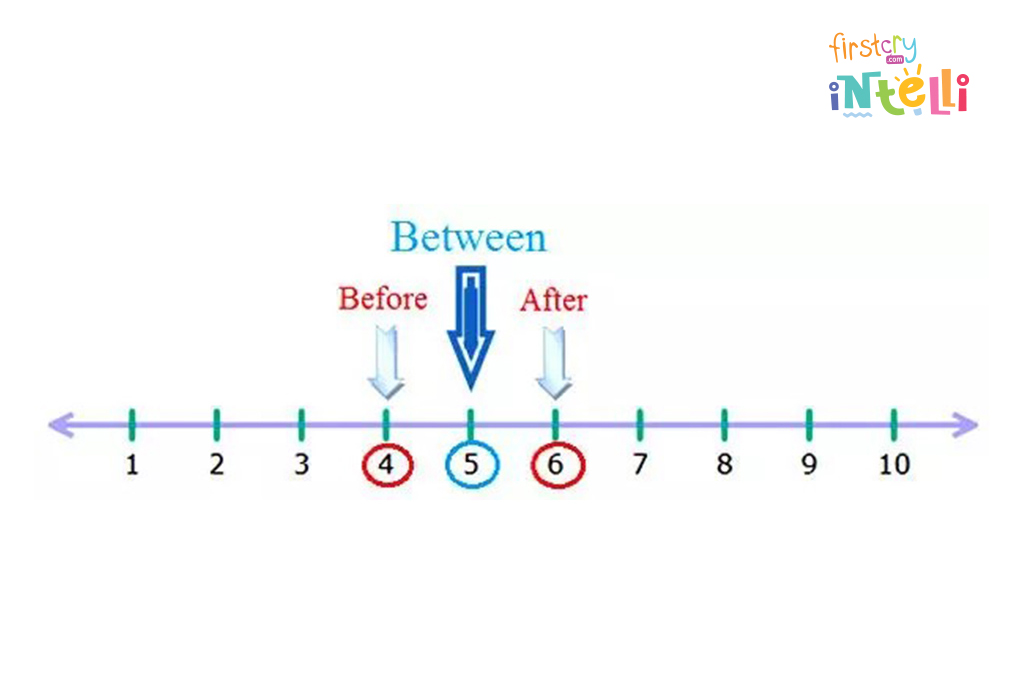#### 1) Before Number

Before number means a number lies left to the given number on the number line. This number is one less than the given number.

• Example 1: What comes before the number 5.

Solution: Count the numbers serially from 1 to 5.
1, 2, 3, 4, 5.
We can see that the number 4 comes before the number 5.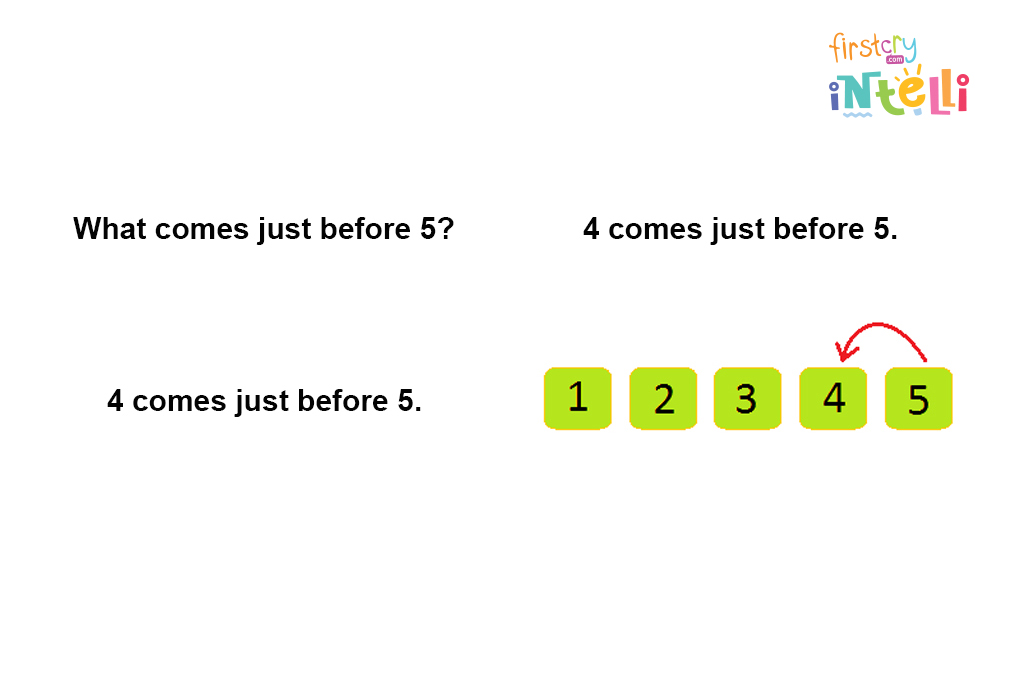• Example 2: What comes before the number 3.

Solution: Count the numbers serially from 1 to 3.
1, 2, 3.
We can see that the number 2 comes before the number 3.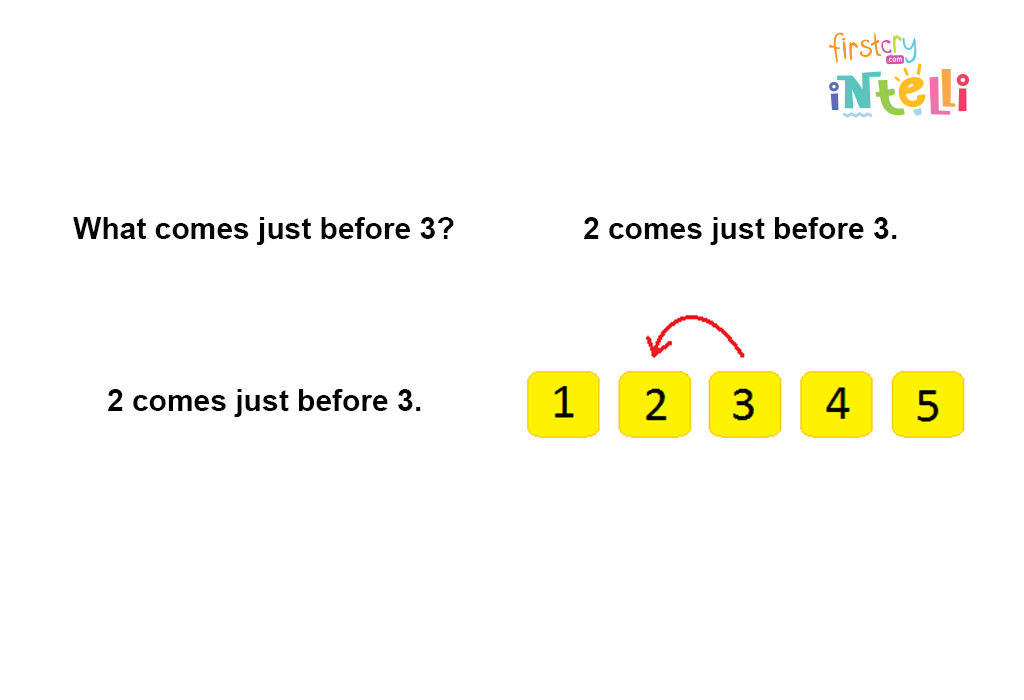#### 2) After Number

After number means a number lies right to the given number on the number line. This number is one more than the given number.

• Example 1: What comes after the number 4.

Solution: Count the numbers serially from 1 to 5.
1, 2, 3, 4, 5.
We can see that the number 5 comes after the number 4.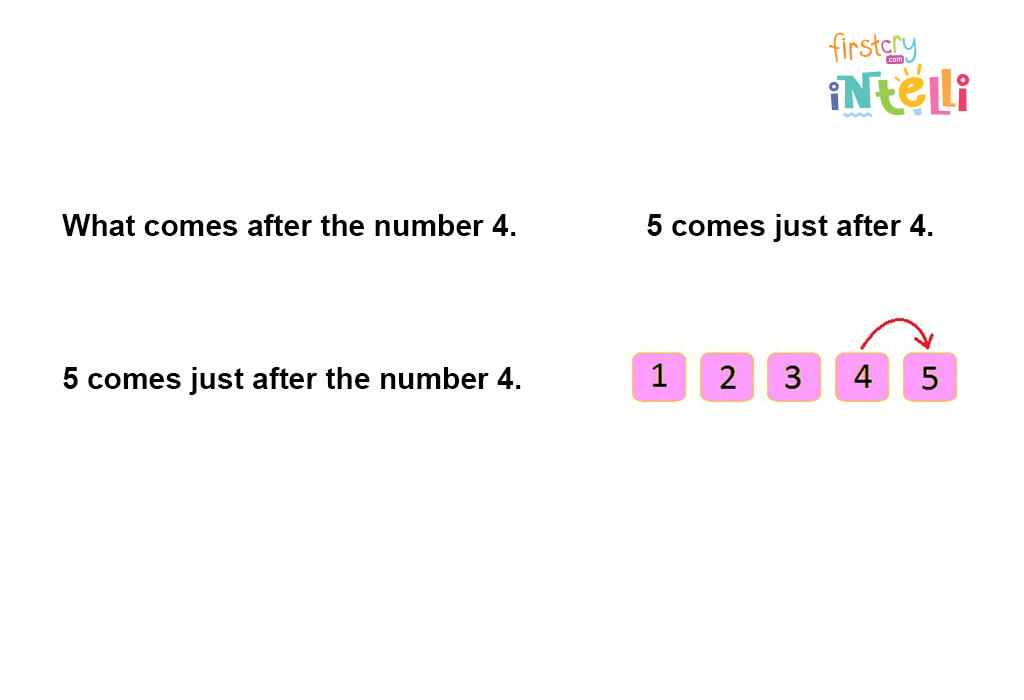• Example 2: What comes after the number 2.

Solution: Count the numbers serially from 1 to 5.
1, 2, 3, 4, 5.
We can see that the number 3 comes after the number 2.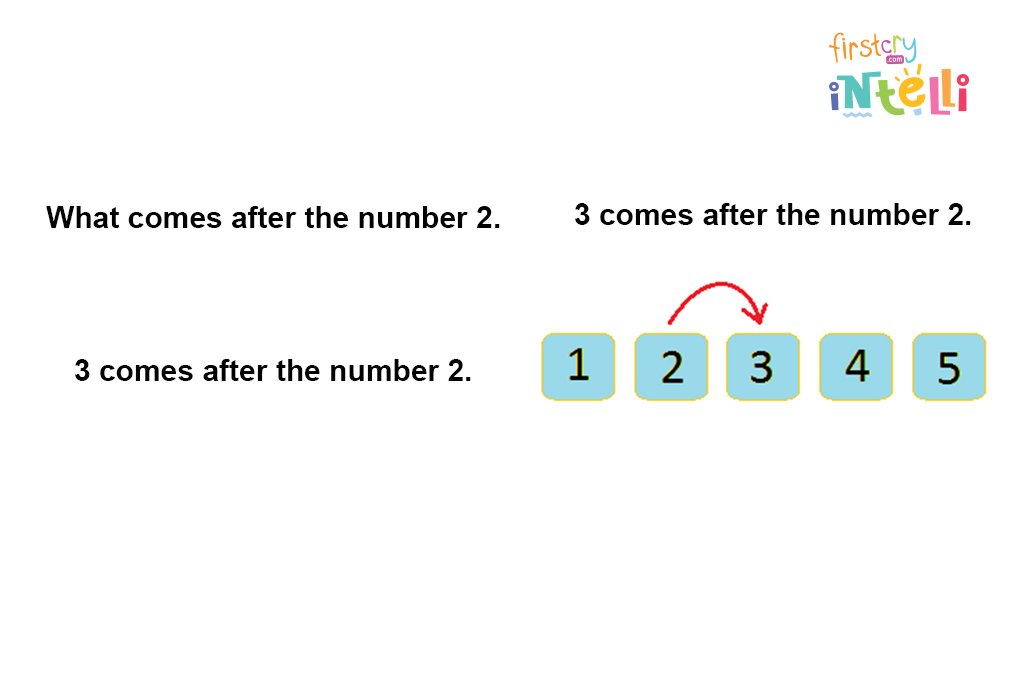#### 3) In Between Number

Between numbers means a number lies in the middle of two numbers. This number is one more than the previous number and one less than the next number. Therefore, it is the middle number between the two numbers.

• Example 1: Find the number between 3 and 5.

Solution: Count the numbers serially.
1, 2, 3, after 3, there is 4, and after 4, there 5.
So, the number between 3 and 5 is 4.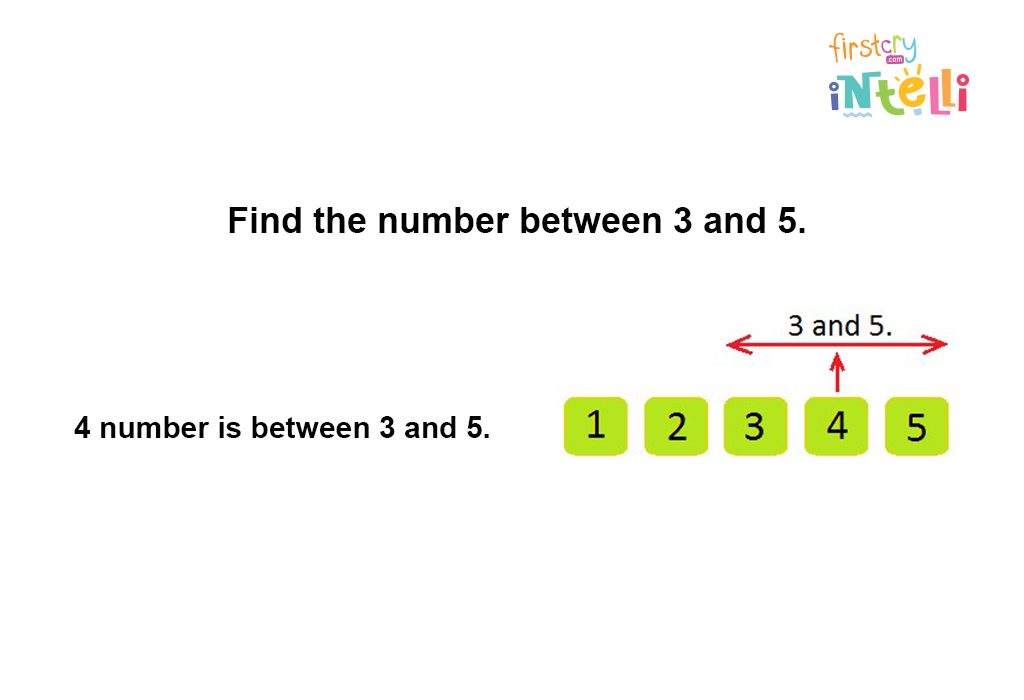• Example 2: Find the number between 2 and 4.

Solution: Count the numbers serially.
1, 2, after 2 there is 3, and after 3, there 4.
So, the number between 2 and 4 is 3.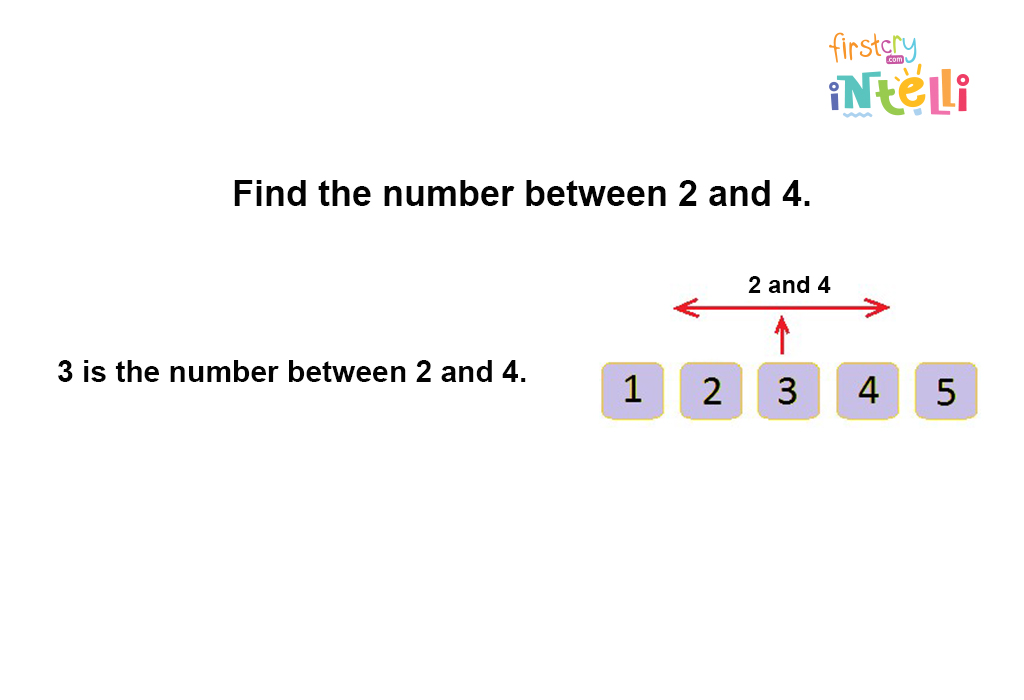###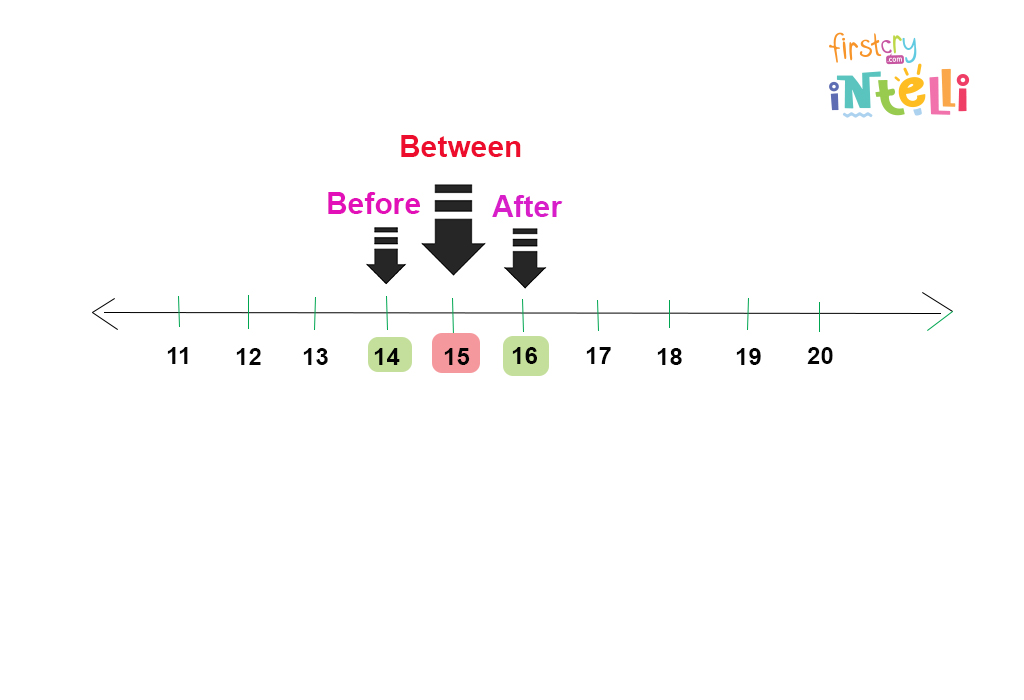As you already know what before, after and between number means, let’s take a look at examples of each for numbers up to 20.

#### 1) Before Number

• Example 1: What number comes before 15?

Solution: 14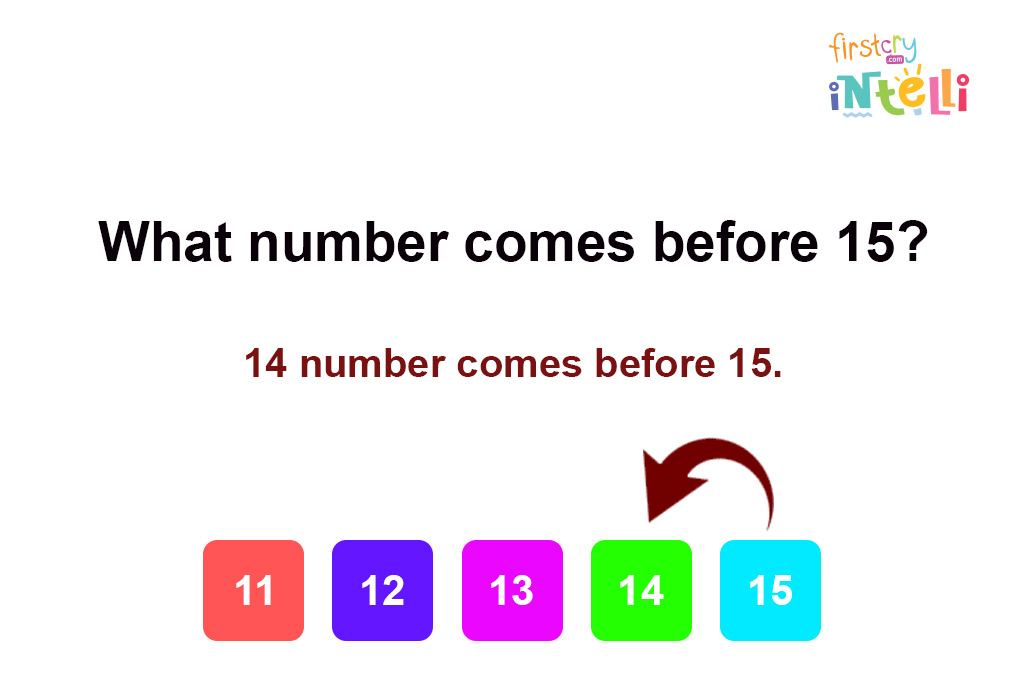• Example 2: What number comes before 17?

Solution: 16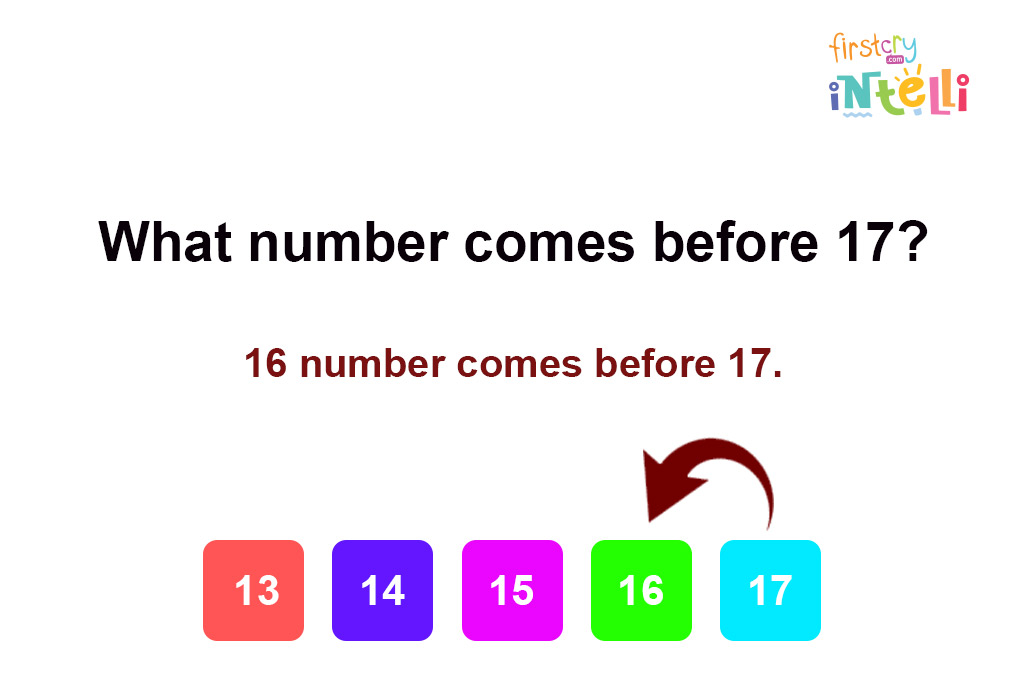#### 2) After Number

• Example 1: What number comes after 15?

Solution: 16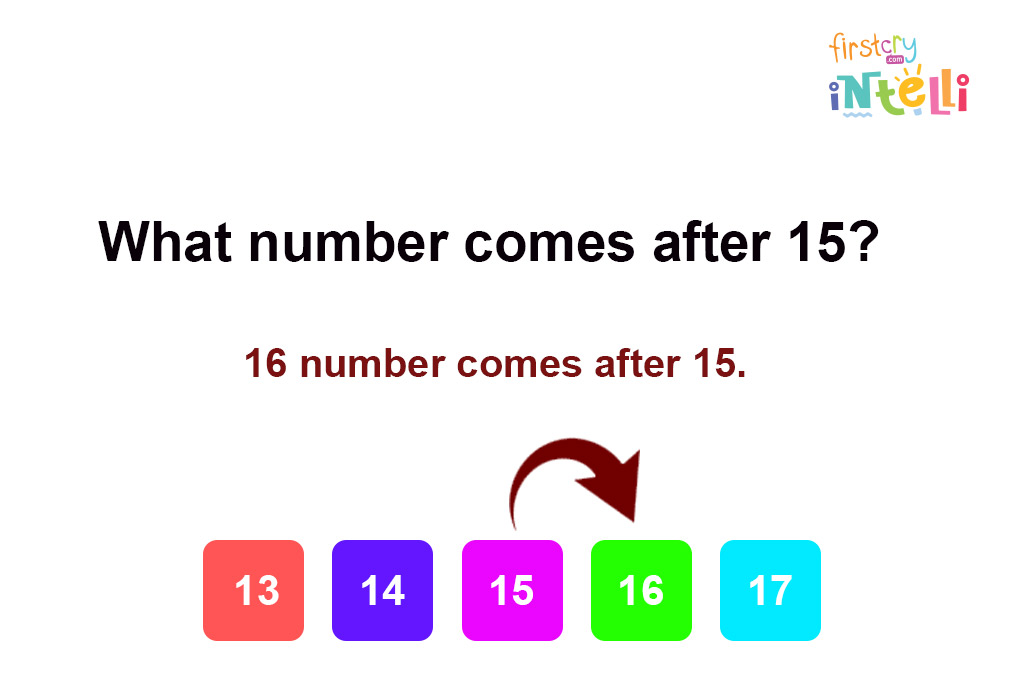• Example 2: What number comes after 17?

Solution: 18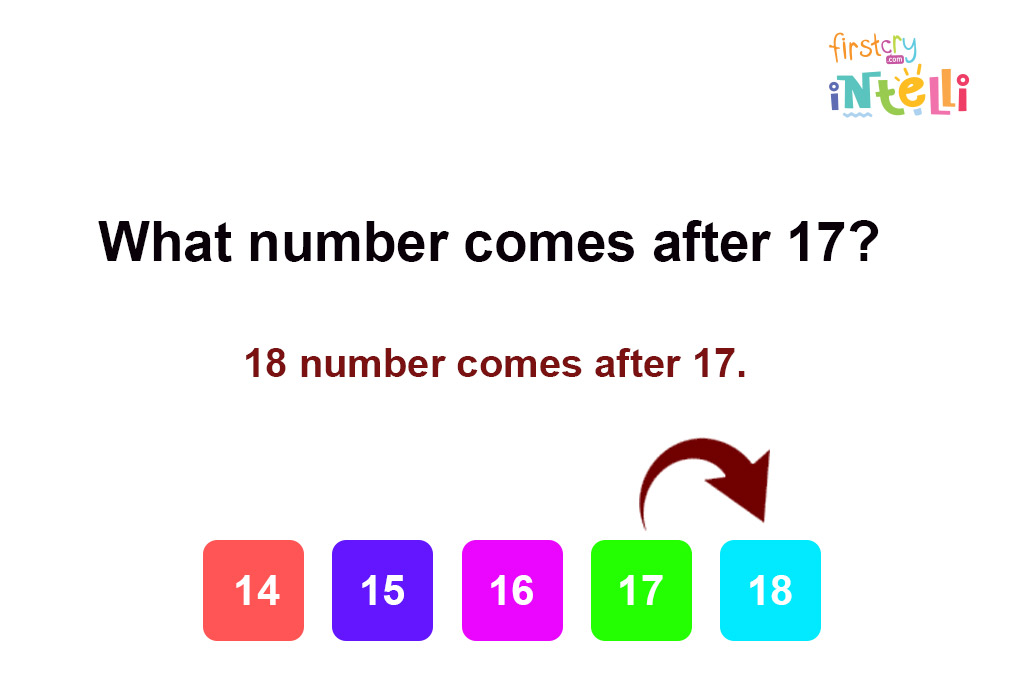#### 3) In Between Number

• Example 1: What number comes between 17 and 19?

Solution: 18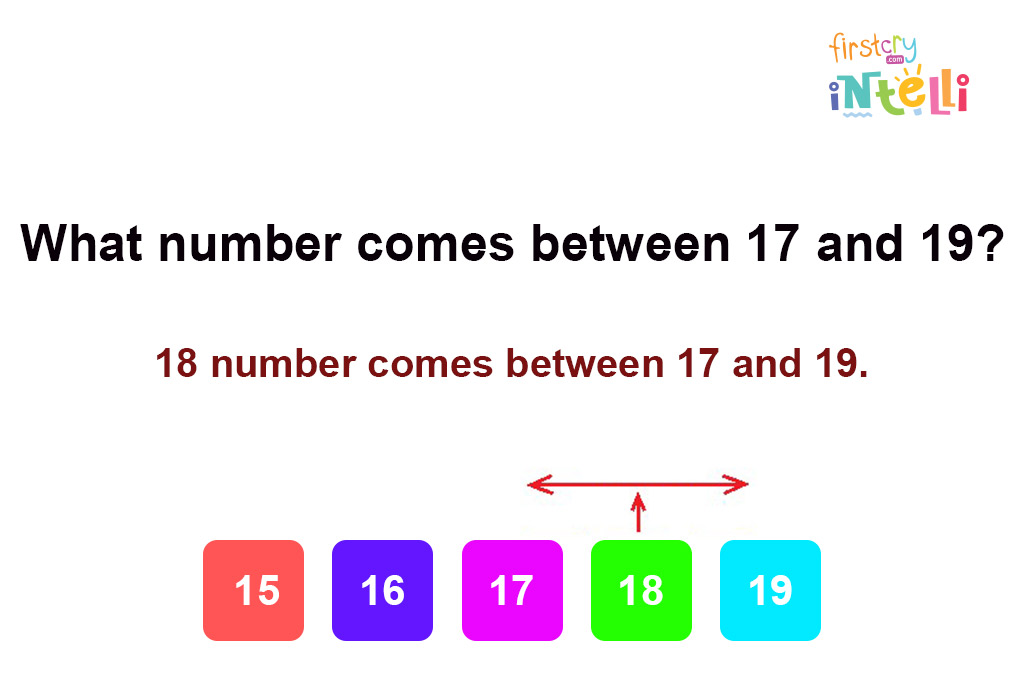• Example 2: What number comes between 12 and 14?

Solution: 13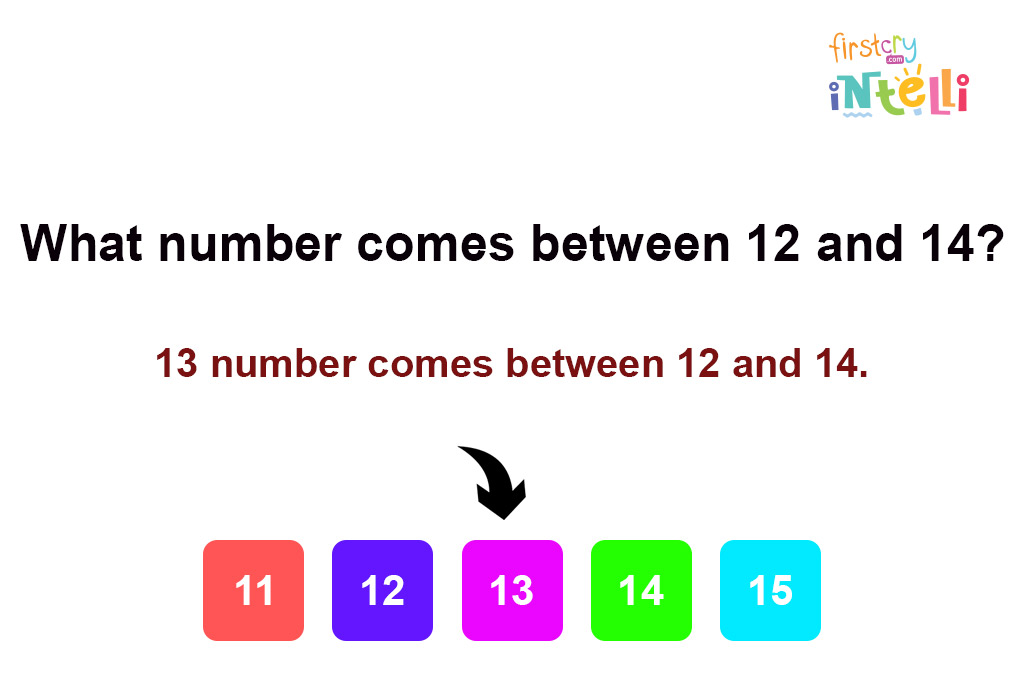## Solved Examples On Before, After And In Between Numbers

In this section, we have covered some solved examples of before, after and in-between numbers for children to understand the concept.

• Example 1: What number comes after 33?

• Example 2: What number comes before 77?

• Example 3: What number comes between 88 and 90?

• Example 4: What number comes after 99?

• Example 5: What number comes between 56 and 58?

Confused about how to teach before and after numbers to kids? Here are some activities on before and after numbers for ukg children.

### 1. Playing Card

Take a deck of playing cards and ask your child to draw one card. Ask them the number on the card and tell them that they have to say the number that comes before and after that number.

### 2. Hopscotch Game

Write down numbers on the floor and ask your child to first identify and then hop to the number you will say. For example, when you will say, “Hop to the number that comes before 5,” your child has to identify that the number is 4 and then hop towards the number.

### 3. Worksheet

On a sheet, write down a few numbers and ask your child to fill in the correct numbers in the blanks. For example, you can use the following numbers for the worksheet:

• 7, _, 9
• _, 10, 11
• 65, 66, _

• 7, 8, 9
• 9, 10, 11
• 65, 66, 67

We hope this article helped your little one to learn about before, after, and in-between concepts in mathematics. Keep practising with the help of the activities shared above, and your child will learn it in no time.Writer, journalist and content creator, she has worked across several publications. She takes a keen interest in travel, food and culture. A new mother, she loves talking about her parenting journey and watching her toddler play, learn and grow. When not working, you will find her watching a movie or web series, or reading a novel, chasing a good story everywhere.
RELATED ARTICLES